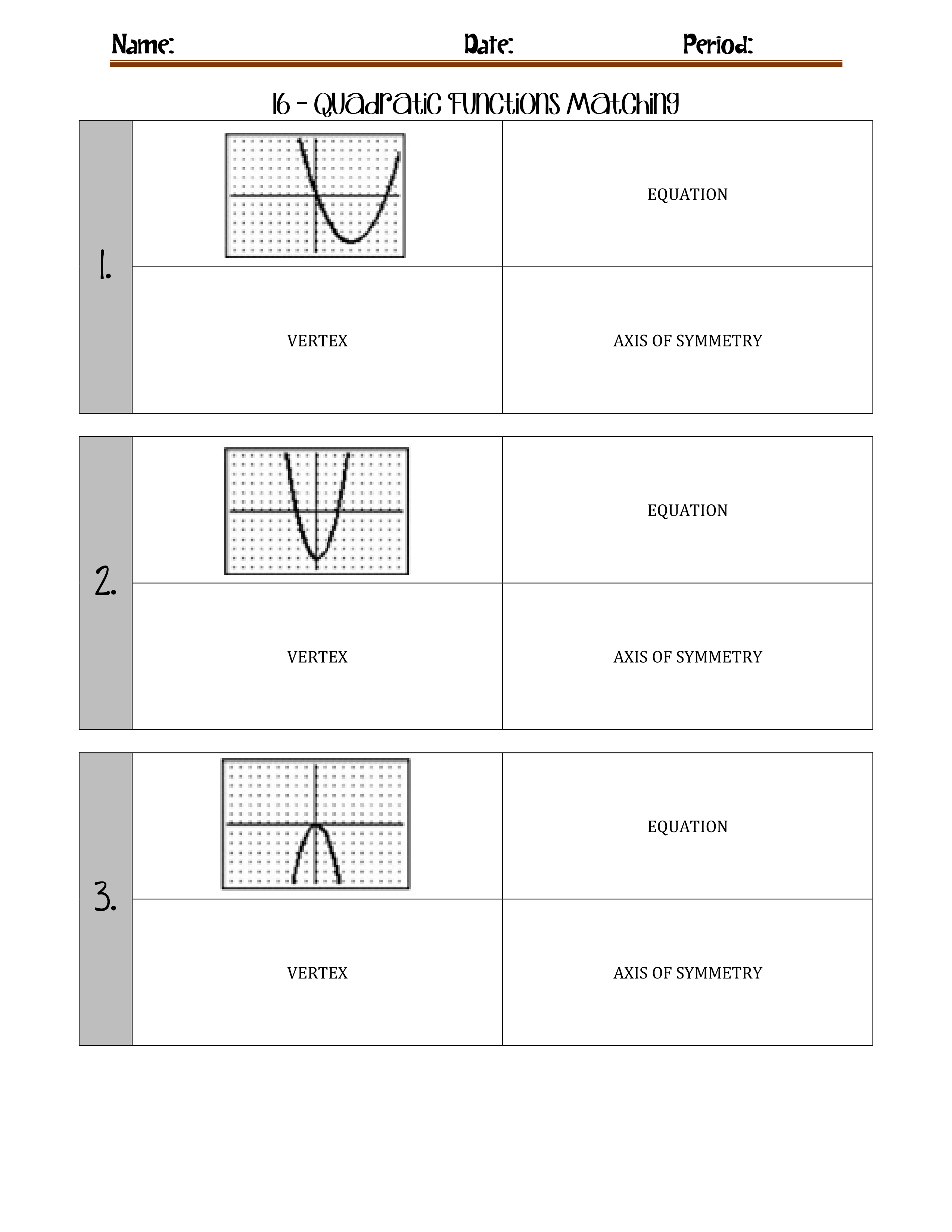Kindergarten
1st
2nd
3rd
4th
5th
6th
7th
8th
9th
10th
11th
12th
Higher Ed
Other
Subjects
ELA
Math
Science
Social Studies
Art
Computer Science
French
German
Music
Physical Education
Spanish
Other
Private Library
16 - Quadratic Functions
starstarstarstarstarstarstarstarstarstar
by Amanda Stolz
| 18 Questions
Note from the author:
zoom in1
1 pt
What is the equation for graph #1?
A

B

C

D

E

F

2
1 pt
What is the vertex for graph #1?
A (0,0)
B (0,-4)
C (4,-5)
D (0,-5)
E (-4,-5)
F (-2,3)
3
1 pt
What is the axis of symmetry of graph #1?
A x=0
B x=-2
C x=4
D x=-4
4
1 pt
What is the equation for graph #2?
A
B
C

D

E

F

5
1 pt
What is the vertex of graph #2?
A (0,0)
B (0,-4)
C (4,-5)
D (0,-5)
E (-4,-5)
F (-2,3)
6
1 pt
What is the Axis of Symmetry for graph #2?
A x=0
B x=-2
C x=4
D x=-4
7
1 pt
What is the equation for graph #3?
A

B

C

D

E

F

8
1 pt
What is the vertex for graph #3?
A (0,0)
B (0,-4)
C (4,-5)
D (0,-5)
E (-4,-5)
F (-2,3)
9
1 pt
What is the Axis of Symmetry for graph #3?
A x=0
B x=-2
C x=4
D x=-4
zoom in10
1 pt
What is the equation for graph #4?
A

B

C

D

E

F

11
1 pt
What is the vertex for graph #4?
A (0,0)
B (0,-4)
C (4,-5)
D (0,-5)
E (-4,-5)
F (-2,3)
12
1 pt
What is the Axis of Symmetry for graph #4?
A x=0
B x=-2
C x=4
D x=-4
13
1 pt
What is the equation for graph #5?
A

B

C

D

E

F
14
1 pt
What is the vertex for graph #5?
A (0,0)
B (0,-4)
C (4,-5)
D (0,-5)
E (-4,-5)
F (-2,3)
15
1 pt
What is the Axis of Symmetry for graph #5?
A x=0
B x=-2
C x=4
D x=-4
16
1 pt
What is the equation of graph #6?
A

B

C

D

E

F

17
1 pt
What is the vertex of graph #6?
A (0,0)
B (0,-4)
C (4,-5)
D (0,-5)
E (-4,-5)
F (-2,3)
18
1 pt
What is the Axis of Symmetry for graph #6?
A x=0
B x=-2
C x=4
D x=-4
Add to my formatives list